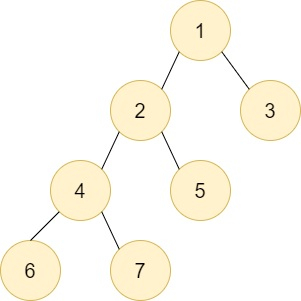# C++ Queries for DFS of a Subtree in a Tree

In this problem we are given a binary tree and we are required to perform dfs from a particular node in which we assume the given node as the root and perform dfs from it.In the above tree suppose we are required to perform DFS from node F

In this tutorial we are going to apply some unorthodox methods so that it will decrease our time complexity substantially and thus we will be able to run this code for higher constraints also.

Approach − In this approach we are not simply going to go the naive way i.e. where we simply apply dfs for every node as it will not work for higher constraints so we try to use some unorthodox methods to avoid getting TLE.

#include <bits/stdc++.h>
using namespace std;
#define N 100000
// Adjacency list to store the
// tree nodes connections
vector<int> v[N];
unordered_map<int, int> mape; // will be used for associating the node with it's index
vector<int> a;
void dfs(int nodesunder[], int child, int parent){ // function for dfs and     precalculation our nodesunder
a.push_back(child); // storing the dfs of our tree
// nodesunder of child subtree
nodesunder[child] = 1;
for (auto it : v[child]) { // performing normal dfs

if (it != parent) { // as we the child can climb up to
//it's parent so we are trying to avoid that as it will become a cycle
dfs(nodesunder, it, child); // recursive call
nodesunder[child] += nodesunder[it]; // storing incrementing the nodesunder
//by the number of nodes under it's children
}
}
}
// Function to print the DFS of subtree of node
void printDFS(int node, int nodesunder[]){
int ind = mape[node]; // index of our node in the dfs array
cout << "The DFS of subtree " << node << ": ";
// print the DFS of subtree
for (int i = ind; i < ind + nodesunder[node]; i++){ // going through dfs array and then
//printing all the nodes under our given node
cout << a[i] << " ";
}
cout << endl;
}
v[x].push_back(y);
v[y].push_back(x);
}
void mark(){ // marking each node with it's index in dfs array
int size = a.size();
// marks the index
for (int i = 0; i < size; i++) {
mape[a[i]] = i;
}
}
int main(){
int n = 7;
// add edges of a tree
// array to store the nodes present under of subtree
// of every node in a tree
int nodesunder[n + 1];
dfs(nodesunder, 1, 0); // generating our nodesunder array
mark(); // marking the indices in map
// Query 1
printDFS(2, nodesunder);
// Query 2
printDFS(4, nodesunder);
return 0;
}

## Output

The DFS of subtree 2: 2 4 6 7 5
The DFS of subtree 4: 4 6 7

## Understanding the code

In this approach we are precalculating the order of dfs and storing it in a vector now when we have precalculated the dfs we also calculate the nodes present under each subtree starting from each node and then we simply traverse form the starting index of then node to all the number of nodes present inside it’s subtree.

## Conclusion

In this tutorial, we solve a problem to solve the Queries for DFS of a subtree in a tree. We also learned C++ program for this problem and the complete approach ( Normal) by which we solved this problem.

We can write the same program in other languages such as C, java, python and other languages. Hope you find this article helpful.